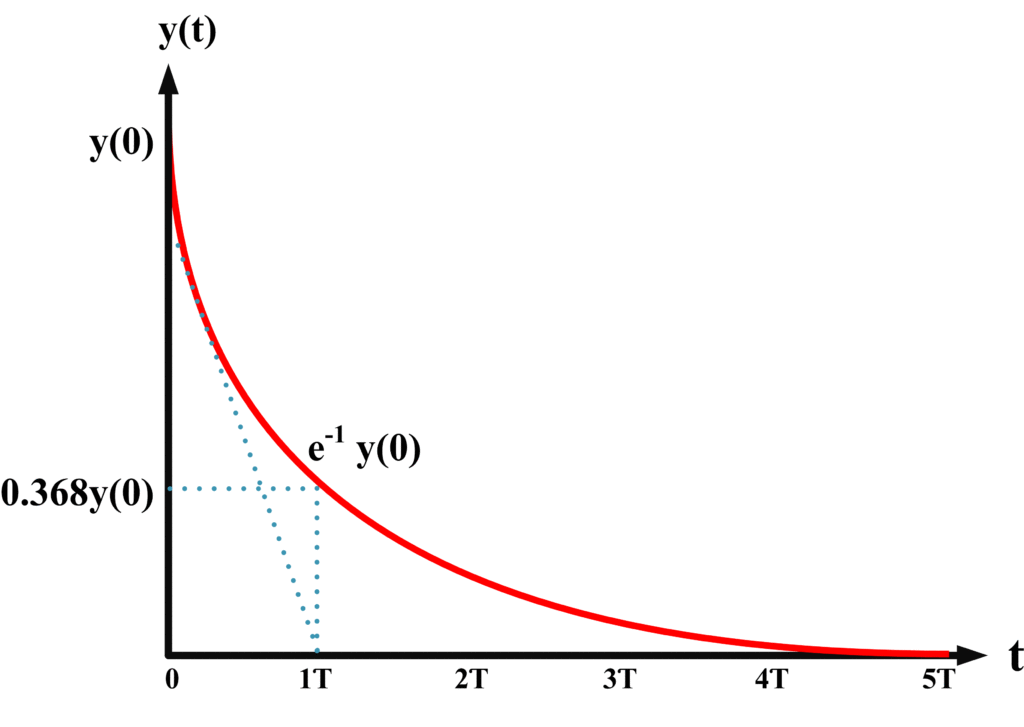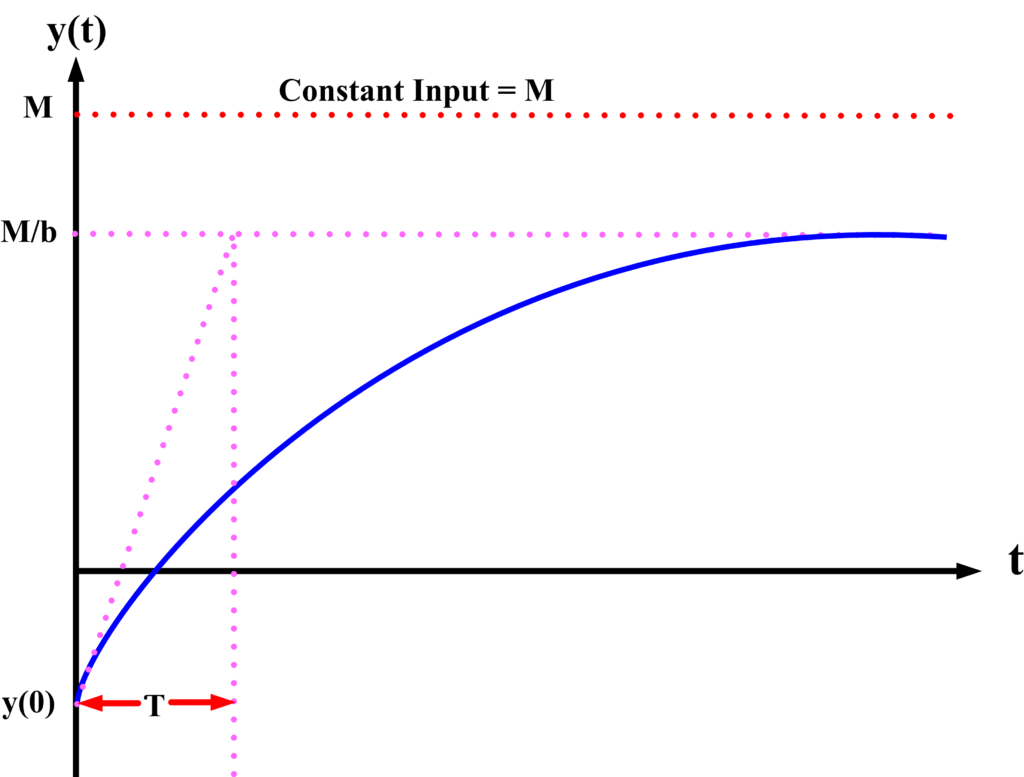# First Order Control System | First Order System Example

Want create site? Find Free WordPress Themes and plugins.

## First Order Control System

First Order System is the one that has only one independent energy storage element. The mathematical expression of first order system can be written in terms of a single variable and its derivative as

$a\frac{dy}{dt}+by=f(t)\text{ (a)}$

The natural or un-driven response for above equation is given as

${{y}_{n}}=y(0){{e}^{-\frac{bt}{a}}}$

Where y(0) is the initial value at time t=0. The above expression can also be written as

${{y}_{n}}=y(0){{e}^{-\frac{t}{\tau }}}\text{ (b)}$

Where t, the time constant of a system, is given by:

$\tau =\frac{a}{b}$

At a time t=τ, the response of the system has decayed to 1/e of its initial value, that is,

${{y}_{n}}=y(0){{e}^{-1}}$

${{y}_{n}}=y(0)\frac{1}{e}$

Or about 36.8% of the initial value. The time constant is one of the main parameters used in characterizing a first-order system. The following figure shows the plot of equation (b):Fig.1: Plot of Equation (b)

When determining the time constant from physical data, it is convenient to construct a tangent to the curve and extend it to the time axis. As shown, the time constant is obtained by measuring the time required for the response to become zero from any point on the curve, assuming the function maintains the rate of change it had at that particular point. This can be shown for point yx as follows. The value for yx is

${{y}_{x}}=y(0){{e}^{-\frac{{{t}_{x}}}{\tau }}}\text{ (c)}$

Where tx is the time when y= yx . the derivative of y from equation b evaluated at t=tx

${{\left. \frac{dy}{dt} \right|}_{t={{t}_{x}}}}=-\frac{y(0)}{\tau }{{e}^{-\frac{{{t}_{x}}}{\tau }}}\text{ (d)}$

From equation (c) and (d) , a time of τ seconds will cause the value of y to change from yx to 0 for any value of yx .

When f(t), the forcing function in equation (a), is a constant step of magnitude M, the complete response is given by

$y=A{{e}^{-\frac{t}{\tau }}}+M/b$

or

$y={{y}_{n}}+{{y}_{f}}$

Where ${{y}_{n}}=A{{e}^{-\frac{t}{\tau }}}$  is the zero-input response and ${{y}_{f}}=M/b$ is the zero-state response (or particular solution). The constant A is determined by the initial condition y(0), and the complete response becomes

$y=M/b+[y(0)-M/b]{{e}^{-\frac{t}{\tau }}}$

As graphed in following figure.Fig.2: Complete System response (natural and forced)

The transfer function of the system represented by equation (a) is given by

$aY(s)s+bY(s)=F(s)$

$T(s)=\frac{Y(s)}{F(s)}=\frac{1}{sa+b}$

Or

$T(s)=\frac{{}^{1}/{}_{b}}{s\frac{a}{b}+1}$

$T(s)=\frac{K}{s\tau +1}$

Where K=1/b is the DC gain of the system and τ=a/b is the system time constant. This form is convenient for representing a first order system in terms of its time constant and steady state gain.

## First Order System Example

A tank in which the level of a liquid is the control variable is frequently encountered a system that can usually be treated as a simple first-order controlled system.

One of the most important groups of first order controlled system is that in which speed is controlled variable. For example, the control of the speed of all type of vehicles, motors, and machines.

Did you find apk for android? You can find new Free Android Games and apps.